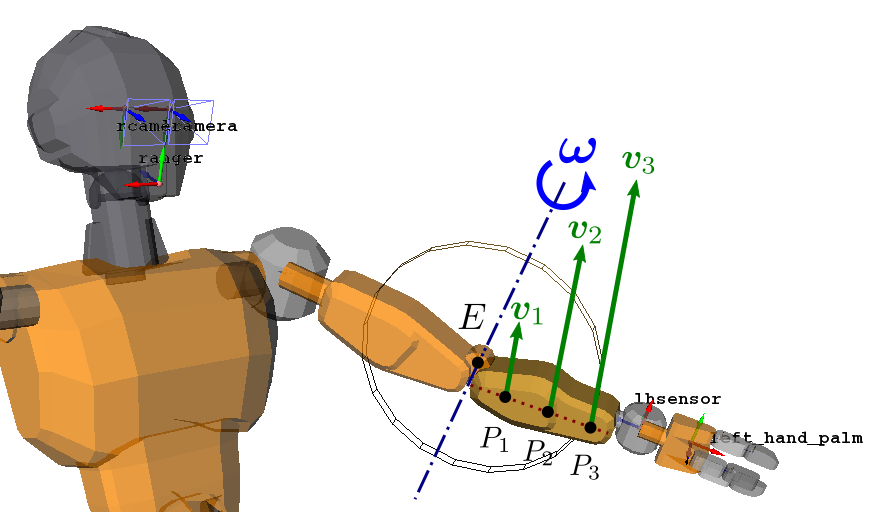# Screw theory

Robots are commonly modeled as a multi-body system, that is, a set of rigid bodies connected by joints. For instance, the mechanical structure of today's humanoid robots is a kinematic tree of cylindrical joints, with the root of the tree at the (rigid body corresponding to the) waist of the robot, and one branch for each limb, left leg, right leg, etc. Motors located in each joint produce torques, which in turn generate a chain of forces between the rigid bodies of the kinematic chain until end-effectors, hands or feet. If the end-effector is free (like a hand in the air), it will perform a pure motion. If it is in contact (like a foot firmly planted on the ground), it will not move directly, but the interaction with the environment will produce contact forces that in turn move the location of the humanoid in space via the Newton-Euler equations of motion. This phenomenon is central to locomotion, and it can be studied, like all rigid-body motions, using the framework of screw theory.

## Screws

The motion of any rigid body is fully described by a mathematical object called a screw, also known as spatial vectors (there may be a subtle difference between these two concepts but I don't understand it for now). A screw $$\bfs_O = (\bfr, \bfm_O)$$ is given by:

• its resultant $$\bfr$$, a vector, and
• its moment $$\bfm_O$$, a vector field over the Euclidean space $$\mathsf{E}^3$$.

The resultant $$\bfr$$ is the same everywhere, but the moment $$\bfm_O$$ depends on the point $$O \in \mathsf{E}^3$$ where it is taken. However, the moment field has a particular structure: from $$\bfm_O$$ and $$\bfr$$, the moment at any other point $$P \in \mathsf{E}^3$$ is given by the Varignon formula:

\begin{equation} \bfm_P \ = \ \bfm_O + \overrightarrow{PO} \times \bfr. \end{equation}

Although the coordinate vector $$\bfs_P$$ of a screw depends on the point $$P$$ where it is taken, the screw itself does not depend on the choice of $$P$$ as a consequence of this formula. There is therefore a distinction to make between the screw itself and its coordinate vector at a given point. A common convention is to denote screws with hats $$\hat\bfs$$ and their coordinates with point subscripts $$\bfs_O$$.

## Twists

Screws are intrinsically related to the motion of rigid bodies in space. Chasles' theorem tells us what these motions consist in:

Chasles' theorem (1830): every spatial displacement is the composition of a rotation about some axis and a translation along the same axis.

Such a displacement (equivalently, such a velocity) is called twist. A twist is a screw $$\bft_O = (\bfomega, \bfv_O)$$ with

• resultant $$\bfomega$$, the angular velocity of the body, and
• moment $$\bfv_O$$, the linear velocity of a given point $$O$$.

The linear velocity represents the translation in Chasles' theorem, while the angular velocity gives the rotation. Consider the case of a pure axial rotation, such as the elbow of a humanoid arm:In the example above, imagine that the robot is rotating its elbow while keeping all other joints fixed. Then, the velocities of the points $$P_1, P_2$$ and $$P_3$$ of the forearm satisfy:

$$\bfv_i = \overrightarrow{P_iE} \times \bfomega,$$

which is Varignon's formula in the special case of a pure rotation where the linear velocity is zero along the rotation axis. This axis is the central axis of the twist.

## Wrenches

A generalized force acting on the body, called wrench, is a screw $$\bfw_O = (\bff, \bftau_O)$$ with

• resultant $$\bff$$, the net force acting on the body, and
• moment $$\bftau_O$$, the net moment of forces acting on the body, taken at a reference point $$O$$.

## Duality

Twists and wrenches live in two dual spaces: the motion space $$\mathsf{M}^6$$ and the force space $$\mathsf{F}^6$$. Duality means that there is a scalar product between twists $$\hat\bft \in \mathsf{M}^6$$ and wrenches $$\hat\bfw \in \mathsf{F}^6$$, defined by:

\begin{equation} \hat\bft \cdot \hat\bfw \ \defeq \ \bft_O \cdot \bfw_O \ = \ \bfv_O \cdot \bff + \bfomega \cdot \bftau_O. \end{equation}

From the Varignon formula, this number does not depend on the point $$O$$ where vector coordinates are taken. When $$\hat\bft$$ and $$\hat\bfw$$ are the twist and wrench acting on a single rigid body, their scalar product represents the instantaneous power of the motion.

## To go further

A reference for this topic is Chapter 2 of Roy Featherstone's Rigid Body Dynamics Algorithms (2010). You can also try Featherstone's previous book, Robot Dynamics Algorithms (1987), where concepts are sometimes more blurry but which I find even more didactic as it follows a single line of thought.

Another excellent read is the seminal 2004 survey paper by Sardain and Bessonnet: Forces Acting on a Biped Robot. Center of Pressure—Zero Moment Point. It uses of the axial representation of screws to gain insight on CoP and ZMP, two characteristic points for contact. This review laid out the foundations for future developments such as the generalization of ZMP support areas to arbitrary contacts.

Pages of this website are under the CC-BY 4.0 license.